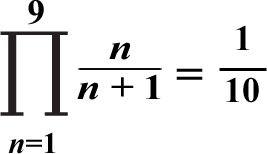# Super Summation 7## Example:1/2 × 2/3 × 3/4 × 4/5 × 5/6 × 6/7 × 7/8 × 8/9 × 9/10 = 1/10

Observe each multiplication step below:

1/2 × 2/3 = 1/3

1/3 × 3/4 = 1/4

1/4 × 4/5 = 1/5

1/5 × 5/6 = 1/6

1/6 × 6/7 = 1/7

1/7 × 7/8 = 1/8

1/8 × 8/9 = 1/9

1/9 × 9/10 = 1/10

No matter what natural number you pick for x, you'll always get 1/(x + 1) as the product summation result!